## The Hamiltonian for the Radiation Field

We now wish to compute the Hamiltonian in terms of the coefficients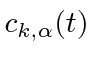. This is an important calculation because we will use the Hamiltonian formalism to do the quantization of the field. We will do the calculation using the covariant notation (while Sakurai outlines an alternate calculation using 3-vectors). We have already calculated the Hamiltonian density for a classical EM field.Now lets compute the basic element of the above formula for our decomposed radiation field.We have all the elements to finish the calculation of the Hamiltonian. Before pulling this all together in a brute force way, its good to realize that almost all the terms will give zero. We see that the derivative of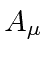is proportional to a 4-vector, say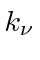and to a polarization vector, say. The dot products of the 4-vectors, either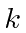with itself orwithare zero. Going back to our expression for the Hamiltonian density, we can eliminate some terms.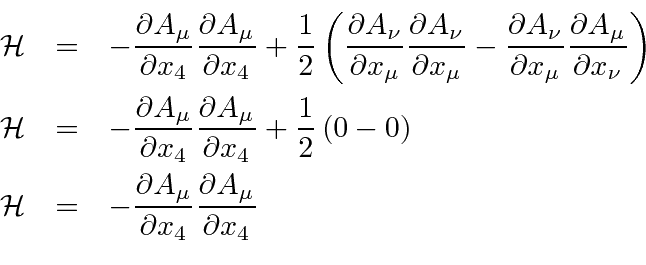The remaining term has a dot product between polarization vectors which will be nonzero if the polarization vectors are the same. (Note that this simplification is possible because we have assumed no sources in the region.)

The total Hamiltonian we are aiming at, is the integral of the Hamiltonian density.When we integrate over the volume only products likewill give a nonzero result. So when we multiply one sum overby another, only the terms with the samewill contribute to the integral, basically because the waves with different wave number are orthogonal.This is the result we will use to quantize the field. We have been careful not to commuteandhere in anticipation of the fact that they do not commute.

It should not be a surprise that the terms that made up the Lagrangian gave a zero contribution because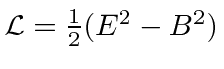and we know that E and B have the same magnitude in a radiation field. (There is one wrinkle we have glossed over; terms with.)

Jim Branson 2013-04-22